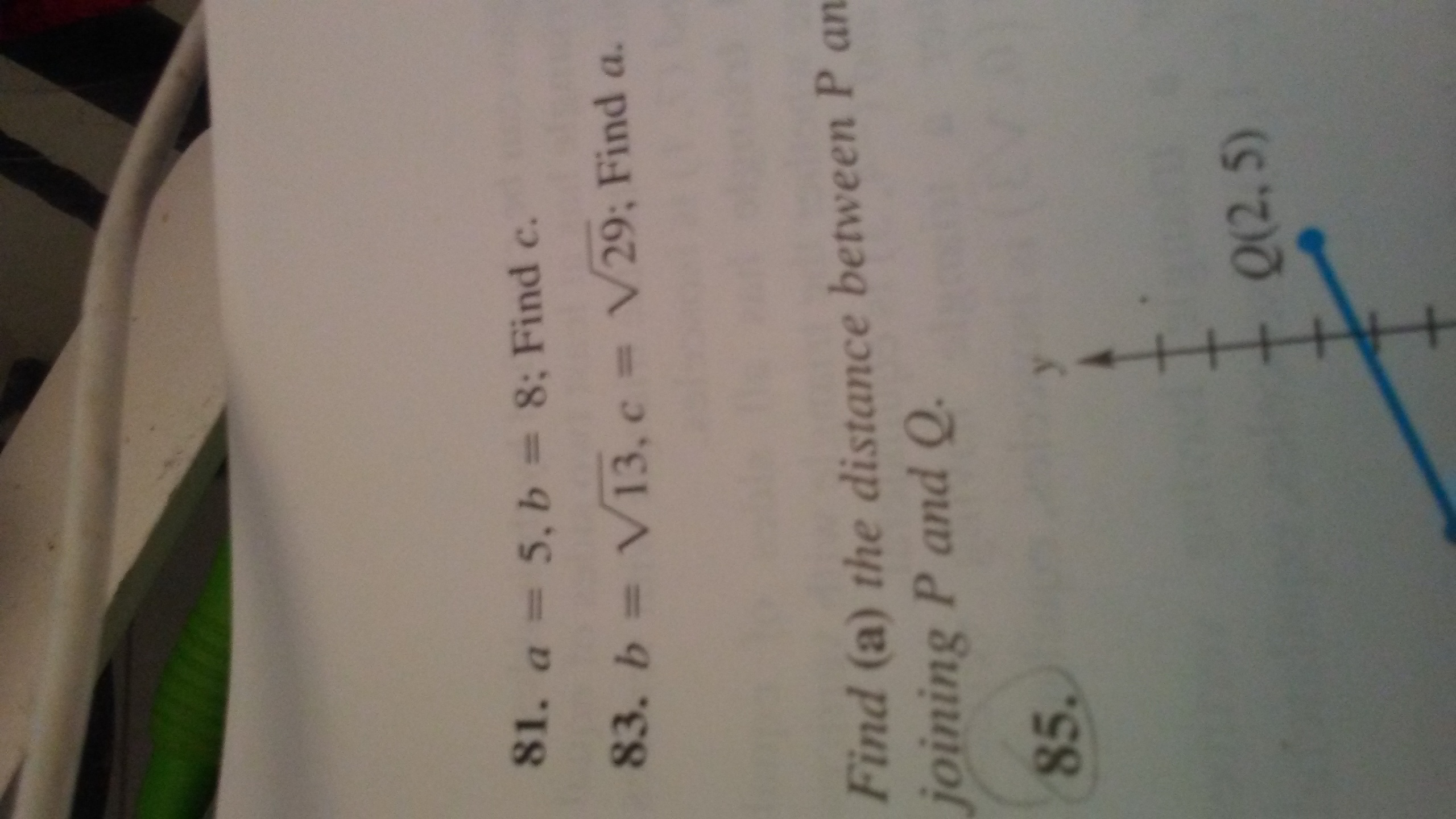# 81. a = 5, b = 8; Find c.83. b= VI3, c = V29; Find a.Find (a) the distance between P anjoining P and Q.85.Q(2,5)

Question
20 views

Find the length of the unknow side of the right triangle. A and B represent the legs and C is the hypotenuse.

Problem 83 is what I need help with.help_outlineImage Transcriptionclose81. a = 5, b = 8; Find c. 83. b= VI3, c = V29; Find a. Find (a) the distance between P an joining P and Q. 85. Q(2,5) fullscreen
check_circle

Step 1

Given:

Step 2

Formula us...

### Want to see the full answer?

See Solution

#### Want to see this answer and more?

Solutions are written by subject experts who are available 24/7. Questions are typically answered within 1 hour.*

See Solution
*Response times may vary by subject and question.
Tagged in

### Algebra